# NCERT Solutions for Class 8 Maths Chapter 6 Exercise 6.1

NCERT Solutions for Class 8 Maths Chapter 6 Exercise 6.1 – Square and Square roots, has been designed by the NCERT to test the knowledge of the student on the following topics :

• Introduction
• Properties of Square Numbers
• Some More Interesting Patterns
2. Numbers between square numbers
4. A sum of consecutive natural numbers
5. Product of two consecutive even or odd natural numbers
6. Some more patterns in square numbers

### NCERT Solutions for Class 8 Maths Chapter 6 Exercise 6.1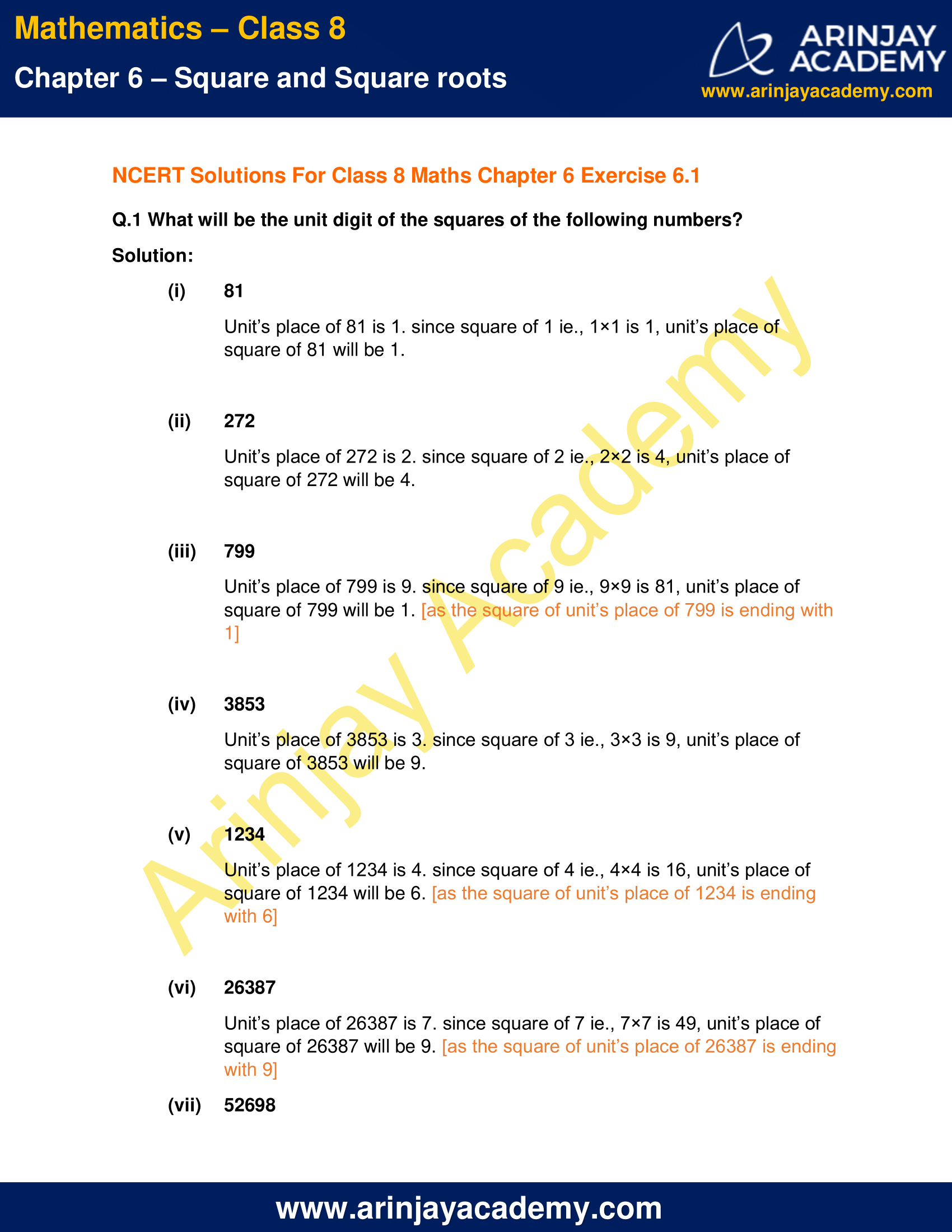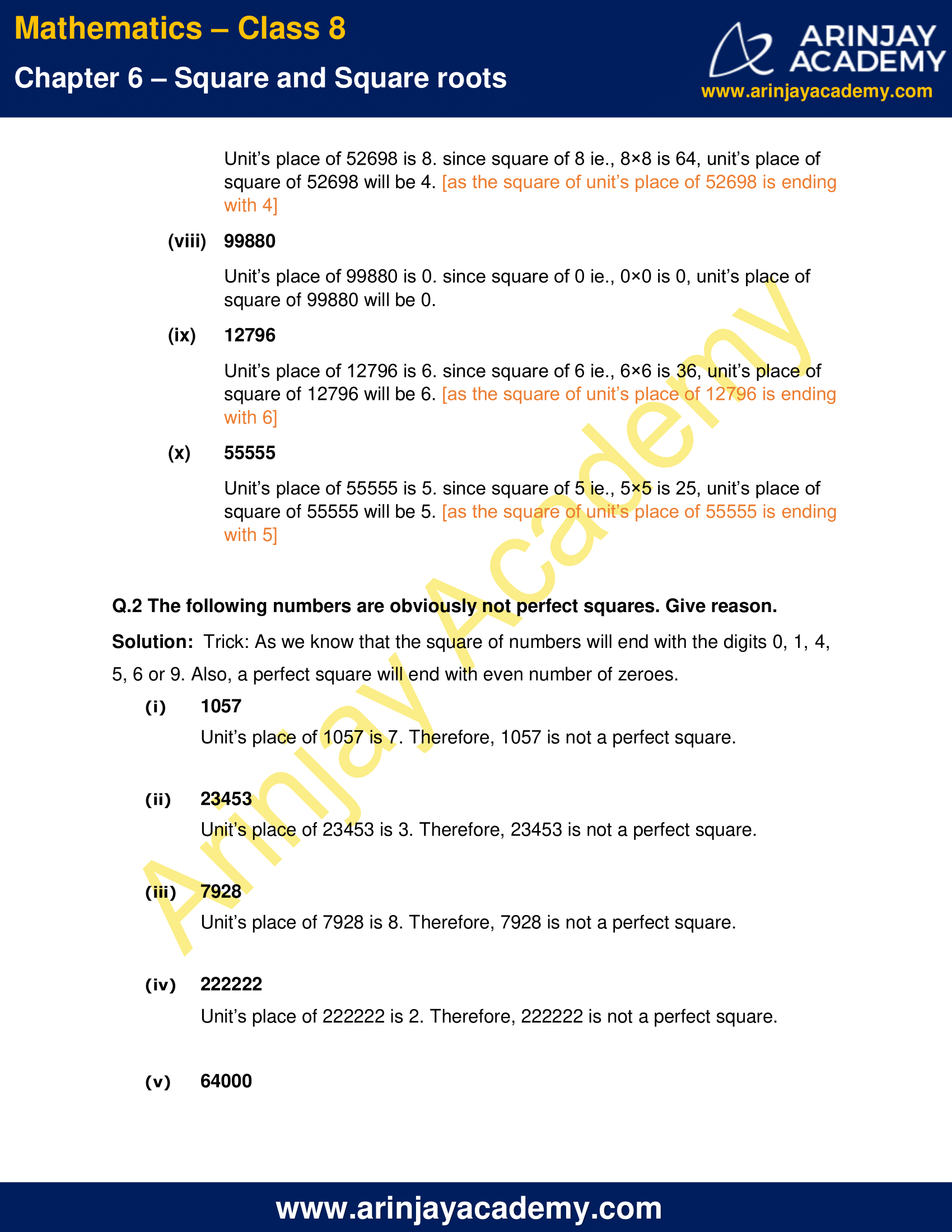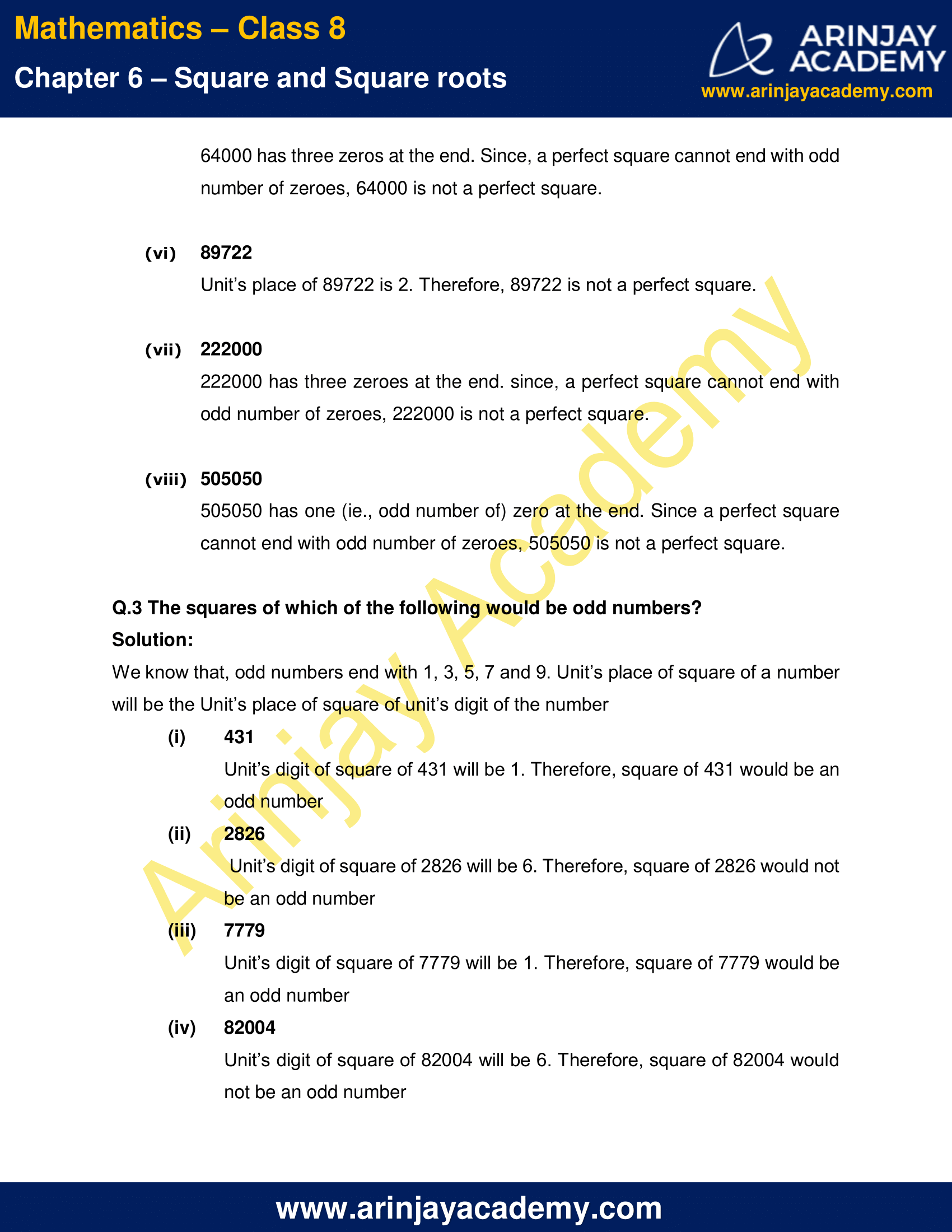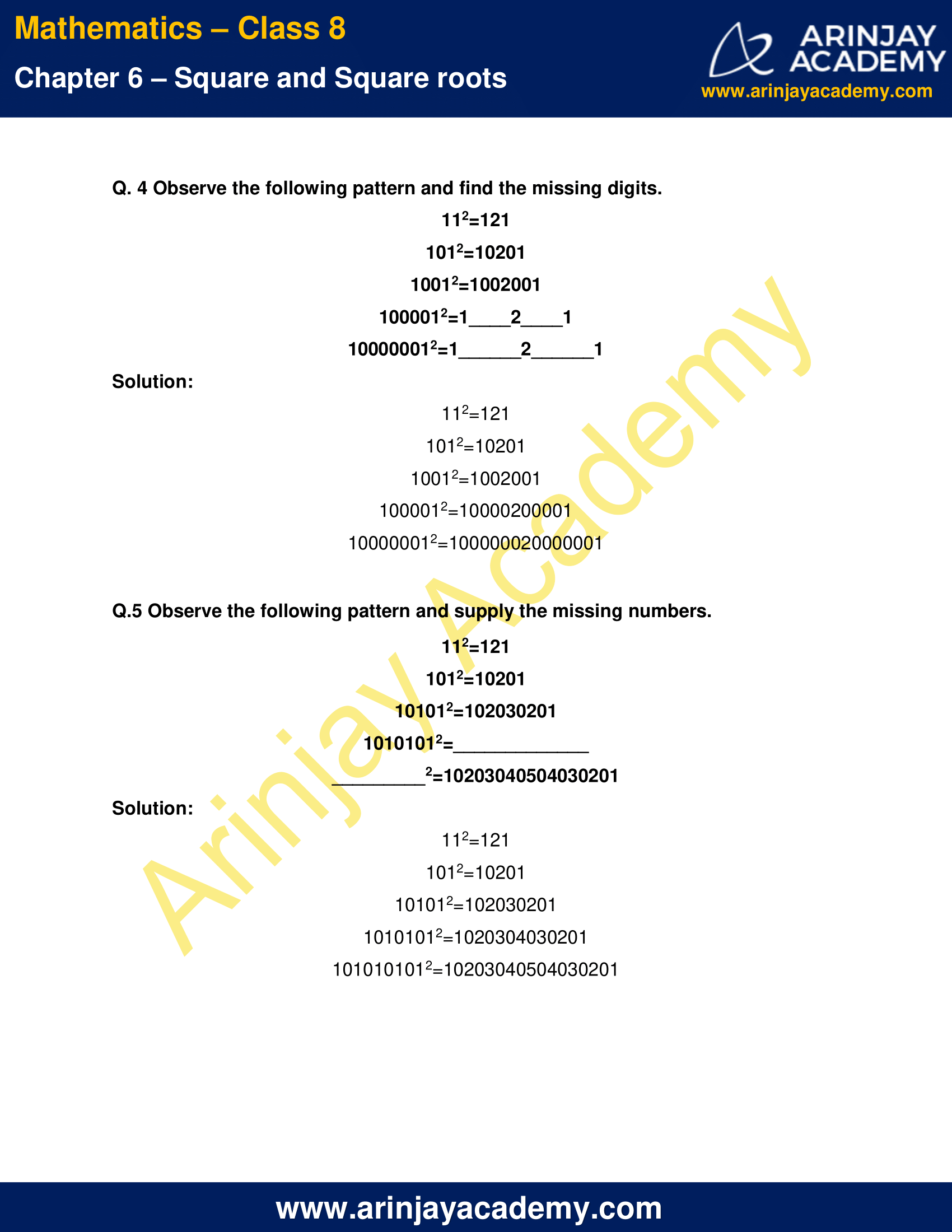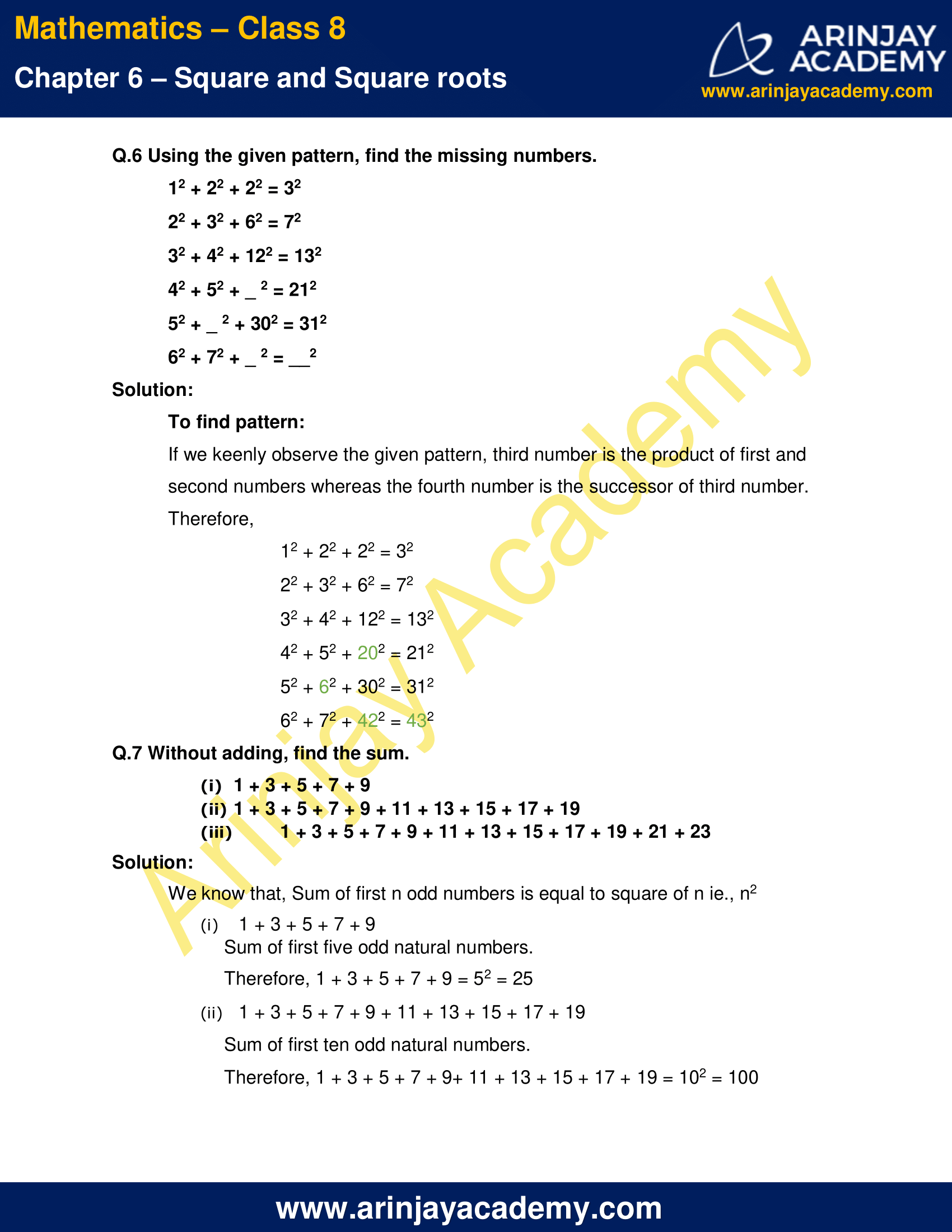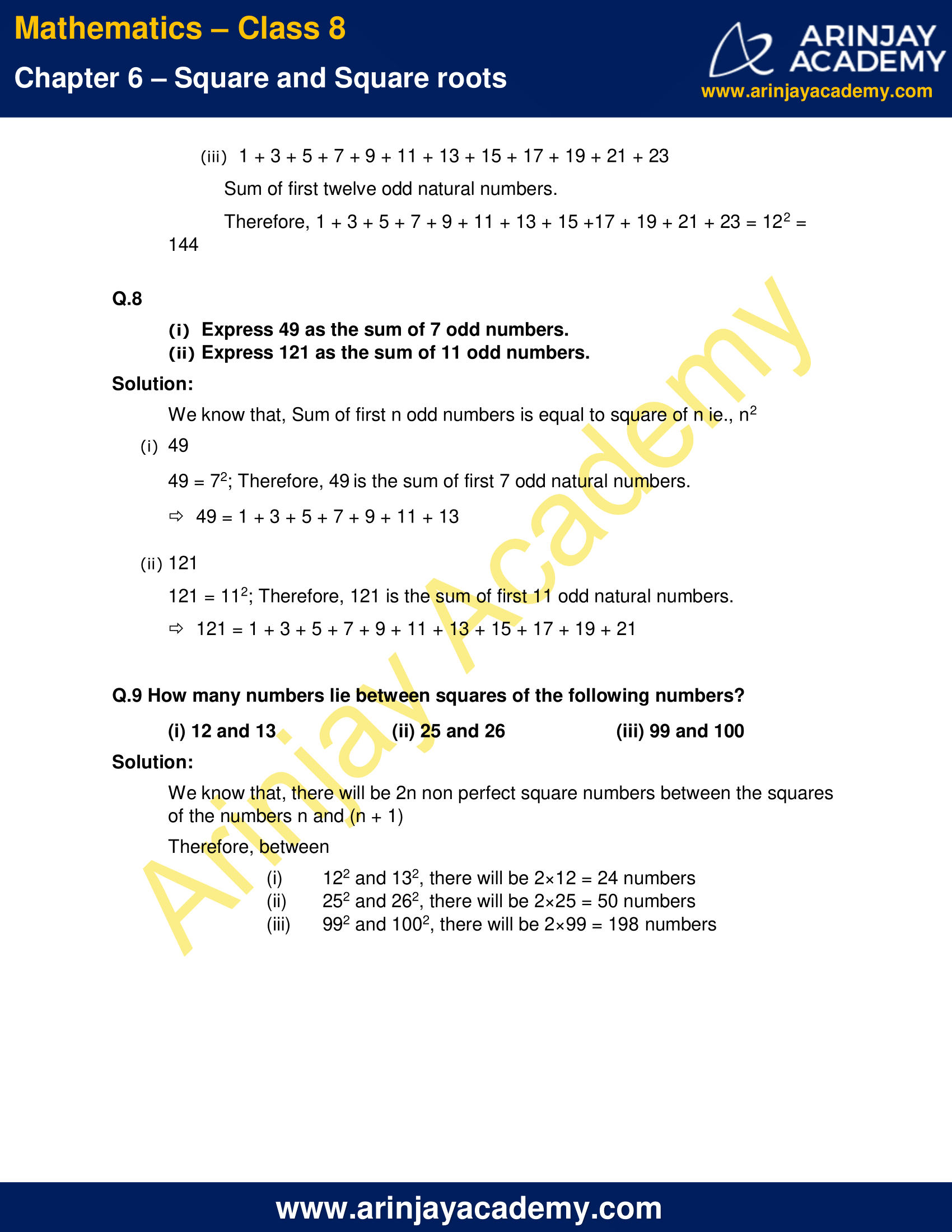NCERT Solutions for Class 8 Maths Chapter 6 Exercise 6.1

Q.1 What will be the unit digit of the squares of the following numbers?

Solution:

(i) 81

Unit’s place of 81 is 1. since square of 1 ie., 1×1 is 1, unit’s place of square of 81 will be 1.

(ii) 272

Unit’s place of 272 is 2. since square of 2 ie., 2×2 is 4, unit’s place of square of 272 will be 4.

(iii) 799

Unit’s place of 799 is 9. since square of 9 ie., 9×9 is 81, unit’s place of square of 799 will be 1. [as the square of unit’s place of 799 is ending with 1]

(iv) 3853

Unit’s place of 3853 is 3. since square of 3 ie., 3×3 is 9, unit’s place of square of 3853 will be 9.

(v) 1234

Unit’s place of 1234 is 4. since square of 4 ie., 4×4 is 16, unit’s place of square of 1234 will be 6. [as the square of unit’s place of 1234 is ending with 6]

(vi) 26387

Unit’s place of 26387 is 7. since square of 7 ie., 7×7 is 49, unit’s place of square of 26387 will be 9. [as the square of unit’s place of 26387 is ending with 9]

(vii) 52698

Unit’s place of 52698 is 8. since square of 8 ie., 8×8 is 64, unit’s place of square of 52698 will be 4. [as the square of unit’s place of 52698 is ending  with 4]

(viii) 99880

Unit’s place of 99880 is 0. since square of 0 ie., 0×0 is 0, unit’s place of square of 99880 will be 0.

(ix) 2796

Unit’s place of 12796 is 6. since square of 6 ie., 6×6 is 36, unit’s place of square of 12796 will be 6. [as the square of unit’s place of 12796 is ending with 6]

(x) 55555

Unit’s place of 55555 is 5. since square of 5 ie., 5×5 is 25, unit’s place of square of 55555 will be 5. [as the square of unit’s place of 55555 is ending  with 5]

Q.2 The following numbers are obviously not perfect squares. Give reason.

Solution:  Trick: As we know that the square of numbers will end with the digits 0, 1, 4, 5, 6 or 9. Also, a perfect square will end with even number of zeroes.

(i) 1057

Unit’s place of 1057 is 7. Therefore, 1057 is not a perfect square.

(ii) 23453

Unit’s place of 23453 is 3. Therefore, 23453 is not a perfect square.

(iii) 7928

Unit’s place of 7928 is 8. Therefore, 7928 is not a perfect square.

(iv) 222222

Unit’s place of 222222 is 2. Therefore, 222222 is not a perfect square.

(v) 64000

64000 has three zeros at the end. Since, a perfect square cannot end with odd number of zeroes, 64000 is not a perfect square.

(vi) 89722

Unit’s place of 89722 is 2. Therefore, 89722 is not a perfect square.

(vii) 222000

222000 has three zeroes at the end. since, a perfect square cannot end with odd number of zeroes, 222000 is not a perfect square.

(viii) 505050

505050 has one (ie., odd number of) zero at the end. Since a perfect square cannot end with odd number of zeroes, 505050 is not a perfect square.

Q.3 The squares of which of the following would be odd numbers?

Solution:

We know that, odd numbers end with 1, 3, 5, 7 and 9. Unit’s place of square of a number will be the Unit’s place of square of unit’s digit of the number

(i) 431

Unit’s digit of square of 431 will be 1. Therefore, square of 431 would be an odd number

(ii) 2826

Unit’s digit of square of 2826 will be 6. Therefore, square of 2826 would not be an odd number

(iii) 7779

Unit’s digit of square of 7779 will be 1. Therefore, square of 7779 would be an odd number

(iv) 82004

Unit’s digit of square of 82004 will be 6. Therefore, square of 82004 would not be an odd number

Q.4  Observe the following pattern and find the missing digits.

112=121

1012=10201

10012=1002001

1000012=1___2___1

100000012=1___2___1

Solution:

112=121

1012=10201

10012=1002001

1000012=10000200001

100000012=100000020000001

Q.5 Observe the following pattern and supply the missing numbers.

112=121

1012=10201

101012=102030201

10101012=_____

___2=10203040504030201

Solution:

112=121

1012=10201

101012=102030201

10101012=1020304030201

1010101012=10203040504030201

Q.6 Using the given pattern, find the missing numbers.

12 + 22 + 22 = 32

22 + 32 + 62 = 72

32 + 42 + 122 = 132

42 + 52 + _ 2 = 212

52 + _ 2 + 302 = 312

62 + 72 + _ 2 = __2

Solution:

To find pattern:

If we keenly observe the given pattern, third number is the product of first and second numbers whereas the fourth number is the successor of third number.

Therefore,

12 + 22 + 22 = 32

22 + 32 + 62 = 72

32 + 42 + 122 = 132

42 + 52 + 202 = 212

52 + 62 + 302 = 312

62 + 72 + 422 = 432

Q.7 Without adding, find the sum.

(i) 1 + 3 + 5 + 7 + 9
(ii) 1 + 3 + 5 + 7 + 9 + 11 + 13 + 15 + 17 + 19
(iii) 1 + 3 + 5 + 7 + 9 + 11 + 13 + 15 + 17 + 19 + 21 + 23

Solution:

We know that, Sum of first n odd numbers is equal to square of n ie., n2

(i) 1 + 3 + 5 + 7 + 9

Sum of first five odd natural numbers.

Therefore, 1 + 3 + 5 + 7 + 9 = 52 = 25

(ii) 1 + 3 + 5 + 7 + 9 + 11 + 13 + 15 + 17 + 19

Sum of first ten odd natural numbers.

Therefore, 1 + 3 + 5 + 7 + 9+ 11 + 13 + 15 + 17 + 19 = 102 = 100

(iii) 1 + 3 + 5 + 7 + 9 + 11 + 13 + 15 + 17 + 19 + 21 + 23

Sum of first twelve odd natural numbers.

Therefore, 1 + 3 + 5 + 7 + 9 + 11 + 13 + 15 +17 + 19 + 21 + 23 = 122 = 144

Q.8

(i) Express 49 as the sum of 7 odd numbers.

(ii) Express 121 as the sum of 11 odd numbers.

Solution:

We know that, Sum of first n odd numbers is equal to square of n ie., n2

(i) 49

49 = 72; Therefore, 49 is the sum of first 7 odd natural numbers.

49 = 1 + 3 + 5 + 7 + 9 + 11 + 13

(ii) 121

121 = 112; Therefore, 121 is the sum of first 11 odd natural numbers.

121 = 1 + 3 + 5 + 7 + 9 + 11 + 13 + 15 + 17 + 19 + 21

Q.9 How many numbers lie between squares of the following numbers?

(i) 12 and 13
(ii) 25 and 26
(iii) 99 and 100

Solution:

We know that, there will be 2n non perfect square numbers between the squares of the numbers n and (n + 1)

Therefore, between

(i) 122 and 132, there will be 2×12 = 24 numbers

(ii) 252 and 262, there will be 2×25 = 50 numbers

(iii) 992 and 1002, there will be 2×99 = 198 numbers

The next Exercise for NCERT Solutions for Class 8 Maths Chapter 6 Exercise 6.2 – Square and Square roots can be accessed by clicking here

Download NCERT Solutions For Class 8 Maths Chapter 6 Exercise 6.1 – Square and Square roots